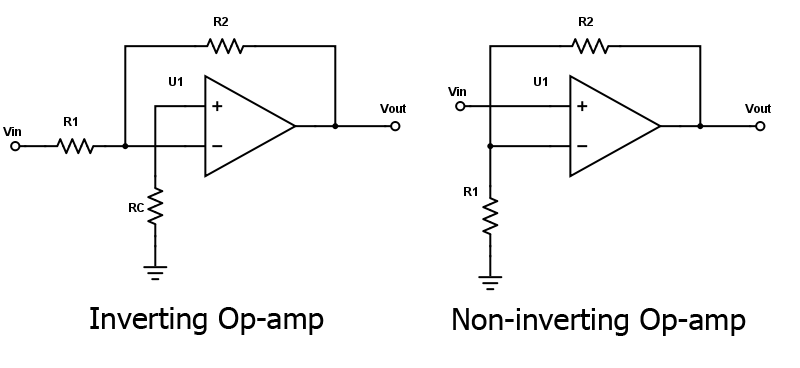# Non-Inverting Op-Amp Resistor Calculator

## This calculator determines the bias and feedback of resistors for a non-inverting Op-Amp

(V)
(KOhms)
(V)
(V)
(V)
(V)

(KOhms)
(KOhms)
(KOhms)

### Overview

A non-inverting operational amplifier (op-amp) amplifies the input signal without inverting its polarity. This tool is designed to compute for the resistors R2, R3 and R4 used in a non-inverting amplifier. The resulting values are in kilo-ohms (kΩ). Note that the non-inverting amplifier circuit used in this calculator has offset control which means you can also provide the offset voltage (V2). If no offset voltage is required, just place zero (0) in the V2 field.### Equations

$$R_{2} = A \frac{R_{1}+R_{4}}{R_{4}R_{1}} - R_{1}$$

$$R_{3} = R_{1}$$

$$R_{4} = A R_{3} \frac{V_{2}}{V_{out2}}$$

$$V_{out1}= A V_{1}$$

$$V_{out2}= V_{out}-V_{out1}$$

### Applications

The operational amplifier is perhaps the most common electronic component and one of the most important. Without operational amplifiers, modern analog circuits would not have been possible. While it is essentially an amplifier, the op-amp is not limited to amplification. It is also used in active filters, analog comparators, and as buffers between two stages of a circuit.

#### Amplifier

The op-amp can be configured as a non-inverting amplifier or as an inverting amplifier. The circuits for these two amplifier configuration is shown below:The gain of the inverting op-amp can be calculated using the formula:

$$A = -\frac{R_{2}}{R_{1}}$$,

while the gain of the non-inverting op-amp is given as:

$$A = 1 + \frac{R_{2}}{R_{1}}$$

To increase the gain, two or more op-amps are cascaded. The overall gain is then the product of the gains of each op-amp (sum if the gain is given in dB).•Johnrey Donaire June 27, 2017
•RK37 January 03, 2018